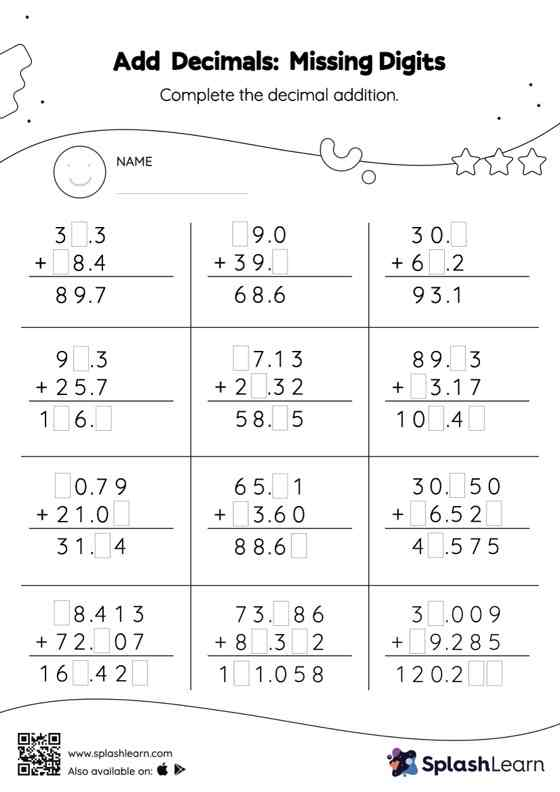# Add Decimals: Missing Digits Worksheet

Home > Add Decimals: Missing DigitsAdd decimals worksheet helps students develop proficiency with addition. Students align the decimal points and use zero as space holders to add decimals. Then they use the relationship between addition and subtraction to find the missing number in add decimals worksheet. In each problem, the numbers are laid out in the vertical format. In this format, pair of digits in each successive place are tackled one by one. This helps students follow a structured approach.